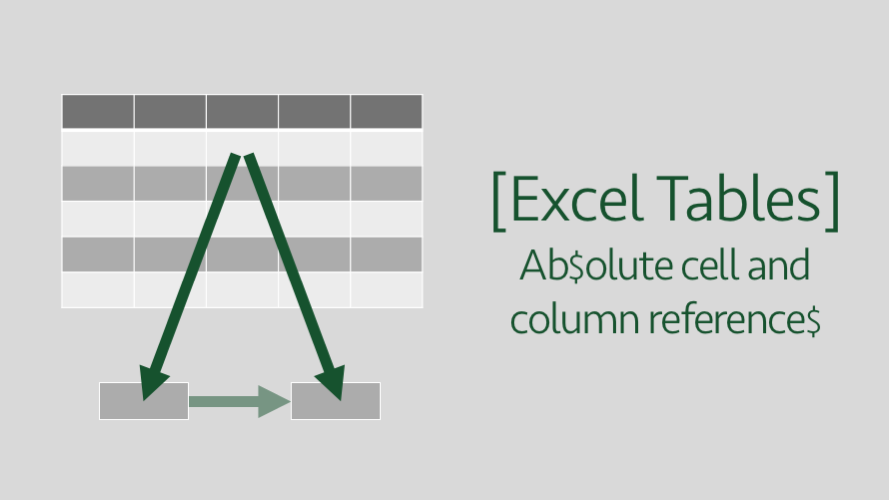# Excel Tables – Absolute cell & column referencesOne of the first things we learn in Excel is the magic of the \$ symbol.  It freezes the row or column, so when copying a formula, the cell reference does not change.  With the introduction of Tables came a different (and more semantic) way to reference cells, called structured references.  However, structured references don’t follow the same principles as the standard A1 style referencing system we usually use.  As a result, the \$ symbol approach won’t work.  But don’t worry, by the end of this post, you will learn that it is possible to switch between relative and absolute references even when using a Table.

The examples in this post all use the following Table.  It shows the costs a Safari Park might incur for owning different types of animals (I went to a zoo with the kids recently, so it’s on my mind, but I’ve made up the data purely for these examples).  The Table name is myTable, whilst it’s not a great name, it will work for this example.## Relative and absolute column references

When using structured references, whole columns are referenced with this syntax:

`tableName[columnName]`

Using the example data, to sum the Total column the formula would be:

`=SUM(myTable[Total])`

If this were dragged or copied to another column, the formula would change automatically.  If copied to the left, it would change to:

`=SUM(myTable[Qty])`

If copied to the right, it would revert to the first column in the table and would change to:

`=SUM(myTable[Animal])`

If using the standard A1 style referencing we could add the \$ signs and change the range from G2:G9 (a relative reference) to \$G\$2:\$G\$9 (an absolute reference).  To achieve the same with a Table, it is necessary to add more square brackets, a colon ( : ) and repeat the column name.

`=SUM(myTable[[Total]:[Total]])`

The difference between an absolute and relative reference is shown in blue above.

If you have been using Tables for a while, you will notice this is the same syntax as when using the mouse to select and reference multiple columns.

`=SUM(myTable[[Food]:[Other]])`

The reference above shows how to sum the columns from Food to Other in the example data.  This means multi-column references selected using the mouse are absolute by default.  To create a relative multi-column reference you’ll need to remove the outer square brackets and repeat the table name, as shown below.

`=SUM(myTable[Food]:myTable[Other])`

Or you could include each column individually within the calculation as shown below, that will achieve the same result.

`=SUM(myTable[Food],myTable[Keepers],myTable[Other])`

## Relative and absolute row references

The syntax to reference a cell in the same row in a Table is as follows.  Please note, the 2nd syntax in each formula is for where the header contains a space or special character.

`=[@Total]    or    =[@[Total Value]]`

Also, if the formula is contained in a cell outside of the Table the table name must also be added:

`=myTable[@Total]    or    =myTable[@[Total Value]`

To make a row reference absolute, the same principles apply as we saw for column references.  It does not matter if the reference is inside or outside the Table, the Table name is required in both circumstances.

`=myTable[@[Total]:[Total]]`

To reference multiple columns the syntax is similar.

`=SUM(myTable[@[Food]:[Other]])`

The reference above shows how to sum the columns from Food to Other from the example data. To create a relative multi-column reference it is necessary to remove the outer square brackets.

`=SUM([@Food]:[@Other])    or    =SUM(myTable[@Food]:myTable[@Other])`

## Absolute references in Headers and Totals

Referencing the Header and Total rows within a Table is slightly different again.

The relative reference for Headers is as shown below:

`=myTable[[#Headers],[Food]]`

To turn the Header reference into an absolute reference add the section marked in below.

`=myTable[[#Headers],[Food]:[Food]]`

If you’ve read this article all the way through, then the multi-column absolute reference will not surprise you.  The formula to count the column headings is shown below.

`=COUNTA(myTable[[#Headers],[Food]:[Other]])`

And the multi-column relative reference will not surprise you either.

`=COUNTA(myTable[[#Headers],[Food]]:myTable[[#Headers],[Other]])`

Totals

The Total row syntax is identical to the Header row noted above, but with [#Totals] in place of [#Headers].

## Conclusion

With a Table, the differences between the various cell references are quite subtle.  An @ or square bracket out of place can be incredibly frustrating to find.  Persistence is key to success, before long it will be second nature.